# GPU-Accelerated Particles with WebGL 2The WebGL 2 specification is based on GL ES 3, and has many new features compared to its predecessor. One of those features is transform feedback. In this post, I'll be exploring how to use it to simulate a lot of particles entirely on the GPU.

NOTE: the demos here use WebGL 2. While it is supported by recent desktop versions of most major browsers, it's still fairly new at the time of this writing. The demos might not work in some mobile browsers. If your browser doesn't support WebGL 2, you should see a placeholder message. In that case, I recommend either updating your current browser, or switching to a recent version of Chrome, Firefox or Opera.

## Particle Systems

Particle systems are great for various visual effects like fireworks, rain, snow, flame, etc. Basically, anything with lots of small bits that fly around and look pretty.

Let's start by defining what we mean when we use the term "particle system" hereafter.

A particle system is a set of independent entities (particles), each of which is fully described by a small set of parameters (same for each particle). The particles appear, disappear and change the values of their parameters depending on time and other factors, according to a well-defined set of simple rules.

The particular (no pun intended) set of parameters describing a particle is chosen by the implementation. You'd want to have obvious mundane things like a particle's position and velocity, but you can also be creative and add other stuff. For example, weight or size.

While we're here, let's decide on a few details about the particle system we're going to build. We're going to have the following parameters for our particles:

• Position - to describe where the particle is
• Velocity - to describe where the particle is headed and how fast
• Age - the number of seconds the particle has been alive
• Life - the number of seconds the particle should stay alive
The following rules will apply:
• Particles obey simple laws of motion (location of the particle after delta seconds equals its current location plus velocity multiplied by delta, velocity is affected by forces acting on the particle at that instance).
• There's one special force affecting all particles at all times. We'll refer to it as "gravity", though this is mere convenience: our "gravity" is tunable by the user, can be set to pull in any direction with any strength, and can be turned off completely.
• Particles cease to exist as soon as they reach a certain age.
• As soon as a particle dies, a new one is born. Total population never exceeds a certain fixed number (chosen by the user).
• Particles do NOT affect each other or the environment (collision detection on the GPU would be interesting to explore, but is outside the scope of this post :-))

## Why GPU Accelerated Particles?

Before we dive into the implementation details though, let's stop for a second and reflect on why what we're about to do makes sense. Let's ask ourselves, what's the most straightforward way to implement a particle system?

The most obvious thing to do, of course, is to go through all the particles every frame, update their parameters according to the rules, and then send the updated positions (and maybe some other parameters) of the particles up to the GPU to be rendered. This works and is simple.

Problems with that solution manifest when you dramatically increase the number of particles in the system (or the number of particle systems themeselves). Suddenly, not only you have to do more work per frame to compute new values of parameters, you also have to deliver a lot more data to the GPU every time you want to draw your particles, which isn't cheap either!

But what if we could run the simulation directly on the GPU? First, we'd be able to harness the parallelism by updating a bunch of particles at a time, instead of one at a time. Second, we wouldn't need to upload the updated data every frame, since it's already right there! Luckily, with transform feedback, running the simulation on GPU is easy to pull off!

## Transform Feedback

As you know, the first programmable stage of the GPU pipeline is the vertex shader. It gets invoked for each vertex, taking as input some per-vertex data, processing it, and spitting out another set of per-vertex data. Usually, the main thing you expect to come out of the vertex shader are the positions of vertices in normalized device coordinates, but you can produce an arbitrary number of additional outputs (often referred to as varyings). The varyings get pushed through the next stages of the pipeline. Transform feedback is a feature that lets you capture some of these varyings into a memory buffer on the GPU, and reuse that data later.

Transform feedback works on both vertex and geometry shader outputs, but since WebGL 2 doesn't support geometry shaders, we'll won't be touching those.

## Writing the Code

### High-Level Overview

• We're going to have two GL programs: the "particle update" program, and the "particle render" program.
• The particle update program will consist of just a vertex shader. It will take as input the current state of particles in the system, and as output, it will produce a buffer containing the updated state of particles after a given elapsed time. Side note: this program will technically have a fragment shader as well (because WebGL 2 won't allow vertex-only programs), but it'll be a no-op that discards all fragments.
• The particle render program will have a vertex shader and a fragment shader. It will take as input the updated state of particles produce a rendering of them.
• In the beginning, we will create two buffers of the same size, big enough to hold the data about the max number of particles possible in our system. One of those we'll designate as the "read" buffer, and the other we'll designate as the "write" buffer.
• We will populate the "read" buffer with the initial state of the particle system.
• On each frame:
• We bind the "read" buffer as input and the "write buffer" as transform feedback buffer. Then we invoke the "particle update" program to write the updated state of particles into the transform feedback buffer.
• Now we bind the "write" buffer as input and invoke the "particle render" program to show the updated state of particles on-screen.
• "Read" and "write" buffers get swapped

### Particle Update - Vertex Shader

Let's start off by writing the vertex shader that implements the particle update step. We'll begin with our uniform inputs. Those are the same for all particles in the system. The meaning of each is explained in a comment.

``````
#version 300 es
precision mediump float;

/* Number of seconds (possibly fractional) that has passed since the last
update step. */
uniform float u_TimeDelta;

/* A texture with just 2 channels (red and green), filled with random values.
This is needed to assign a random direction to newly born particles. */
uniform sampler2D u_RgNoise;

/* This is the gravity vector. It's a force that affects all particles all the
time.*/
uniform vec2 u_Gravity;

/* This is the point from which all newborn particles start their movement. */
uniform vec2 u_Origin;

/* Theta is the angle between the vector (1, 0) and a newborn particle's
velocity vector. By setting u_MinTheta and u_MaxTheta, we can restrict it
to be in a certain range to achieve a directed "cone" of particles.
To emit particles in all directions, set these to -PI and PI. */
uniform float u_MinTheta;
uniform float u_MaxTheta;

/* The min and max values of the (scalar!) speed assigned to a newborn
particle.*/
uniform float u_MinSpeed;
uniform float u_MaxSpeed;
```
```

Side note: as you can see, I am using a noise texture here, as a source of randomness. It is possible to do without a texture at all. There are ways to generate random numbers on the GPU.

Now, let's move on to our inputs and outputs:

``````
/* Inputs. These reflect the state of a single particle before the update. */

/* Where the particle is. */
in vec2 i_Position;

/* Age of the particle in seconds. */
in float i_Age;

/* How long this particle is supposed to live. */
in float i_Life;

/* Which direction it is moving, and how fast. */
in vec2 i_Velocity;

/* Outputs. These mirror the inputs. These values will be captured
into our transform feedback buffer! */
out vec2 v_Position;
out float v_Age;
out float v_Life;
out vec2 v_Velocity;
```
```

The above should be fairly obvious. We have a one-to-one mapping between vertices and particles: every invocation of the vertex shader has to update just one corresponding particle. Let's see how it's actually done:

``````
void main() {
if (i_Age >= i_Life) {
/* Particle has exceeded its lifetime! Time to spawn a new one
in place of the old one, in accordance with our rules.*/

/* First, choose where to sample the random texture. I do it here
based on particle ID. It means that basically, you're going to
get the same initial random values for a given particle. The result
still looks good. I suppose you could get fancier, and sample
based on particle ID *and* time, or even have a texture where values
are not-so-random, to control the pattern of generation. */
ivec2 noise_coord = ivec2(gl_VertexID % 512, gl_VertexID / 512);

/* Get the pair of random values. */
vec2 rand = texelFetch(u_RgNoise, noise_coord, 0).rg;

/* Decide the direction of the particle based on the first random value.
The direction is determined by the angle theta that its vector makes
with the vector (1, 0).*/
float theta = u_MinTheta + rand.r*(u_MaxTheta - u_MinTheta);

/* Derive the x and y components of the direction unit vector.
This is just basic trig. */
float x = cos(theta);
float y = sin(theta);

/* Return the particle to origin. */
v_Position = u_Origin;

/* It's new, so age must be set accordingly.*/
v_Age = 0.0;
v_Life = i_Life;

/* Generate final velocity vector. We use the second random value here
to randomize speed. */
v_Velocity =
vec2(x, y) * (u_MinSpeed + rand.g * (u_MaxSpeed - u_MinSpeed));

} else {
/* Update parameters according to our simple rules.*/
v_Position = i_Position + i_Velocity * u_TimeDelta;
v_Age = i_Age + u_TimeDelta;
v_Life = i_Life;
v_Velocity = i_Velocity + u_Gravity * u_TimeDelta;
}
}
```
```

That's it for the particle update shader. We'll make some small modifications to it later, but the main logic is there.

### Rendering the Particles

Now, let's write a couple of simple shaders to actually visualize the output of the previous step. This is going to be very basic for the time being, but we will add to it later.

Here we go, vertex shader for visualizing particles, version 1:

``````
#version 300 es
precision mediump float;

in vec2 i_Position;
in float i_Age;
in float i_Life;
in vec2 i_Velocity;

void main() {
gl_PointSize = 1.0;
gl_Position = vec4(i_Position, 0.0, 1.0);
}
```
```

As you can see, it is intended to draw points primitives. For now, it's only using the position attribute, but this is going to change later.

And here's the corresponding fragment shader, also super simple for now:

``````
#version 300 es
precision mediump float;

out vec4 o_FragColor;

void main() {
o_FragColor = vec4(1.0);
}
```
```

Basically, this pair of shaders makes particles appear on screen as white dots. Like I said, we'll make it a bit fancier later :-)

### The JavaScript Code

Before we can finally see our shaders in action, we have to write a bit of javascript code.

``````
<script type = "text/x-fragment-shader" id = "particle-render-frag">
// ... shader code goes here...
</script>
```
```

Here is a pair of functions to load shaders from "script" tags, compile them and link them into a program. I would normally skip this as boilerplate, but it contains a little bit of detail that is relevant to our interests.

``````
var i = 0;
/* skip whitespace to avoid glsl compiler complaining about
#version not being on the first line*/
if (!compile_status) {
throw "Could not compile shader \"" +
"\" \n" +
error_message;
}
}

/* Creates an OpenGL program object.
`gl' shall be a WebGL 2 context.
`shader_list' shall be a list of objects, each of which have a `name'
and `type' properties. `name' will be used to locate the script tag
`transform_feedback_varyings' shall be a list of varying that need to be
captured into a transform feedback buffer.*/
var program = gl.createProgram();
for (var i = 0; i < shader_list.length; i++) {
}

/* Specify varyings that we want to be captured in the transform
feedback buffer. */
if (transform_feedback_varyings != null) {
gl.transformFeedbackVaryings(
program,
transform_feedback_varyings,
gl.INTERLEAVED_ATTRIBS)
}

var error_message = gl.getProgramInfoLog(program);
throw "Could not link program.\n" + error_message;
}
return program;
}
```
```

I want you to pay attention to the third parameter of `createGLProgram`. As I mentioned earlier, transform feedback lets us capture the values of the varyings that a vertex shader outputs. However, we must specify the varyings that we want to capture before we link the program. This is done through a call to `transformFeedbackVaryings`. When calling that function, you have to specify the program you're dealing with, the list of captured varyings, and the preferred way of capture (capture them interleaved into a single buffer, or capture each one into a separate buffer). In this case we're using interleaved capture mode.

Next, I am going to introduce a couple of helper functions that we're going to use during the initialization process of our particle system.

``````
function randomRGData(size_x, size_y) {
var d = [];
for (var i = 0; i < size_x * size_y; ++i) {
d.push(Math.random() * 255.0);
d.push(Math.random() * 255.0);
}
return new Uint8Array(d);
}

function initialParticleData(num_parts, min_age, max_age) {
var data = [];
for (var i = 0; i < num_parts; ++i) {
// position
data.push(0.0);
data.push(0.0);

var life = min_age + Math.random() * (max_age - min_age);
// set age to max. life + 1 to ensure the particle gets initialized
// on first invocation of particle update shader
data.push(life + 1);
data.push(life);

// velocity
data.push(0.0);
data.push(0.0);
}
return data;
}
```
```

The first one, `randomRGData`, simply generates data for a random 2-channel texture that is going to be used in the particle update shader.

The second one, `initialParticleData`, generates the data representing the initial state of the particle system. This will get sent to the GPU once at the beginning, and from there it will be updated by the update shader.

The next portion of code does the setup of our particle system. It's a bit long, but I added comments for you. Don't skip reading them! Also, you should know about vertex array objects to understand this part, since I'm using them here.

``````
/*
This is a helper function used by the main initialization function.
It sets up a vertex array object based on the given buffers and attributes
they contain.
If you're familiar with VAOs, following this should be easy.
*/
function setupParticleBufferVAO(gl, buffers, vao) {
gl.bindVertexArray(vao);
for (var i = 0; i < buffers.length; i++) {
var buffer = buffers[i];
gl.bindBuffer(gl.ARRAY_BUFFER, buffer.buffer_object);
var offset = 0;
for (var attrib_name in buffer.attribs) {
if (buffer.attribs.hasOwnProperty(attrib_name)) {
/* Set up vertex attribute pointers for attributes that are stored in this buffer. */
var attrib_desc = buffer.attribs[attrib_name];
gl.enableVertexAttribArray(attrib_desc.location);
gl.vertexAttribPointer(
attrib_desc.location,
attrib_desc.num_components,
attrib_desc.type,
false,
buffer.stride,
offset);
/* we're only dealing with types of 4 byte size in this demo, unhardcode if necessary */
var type_size = 4;

/* Note that we're cheating a little bit here: if the buffer has some irrelevant data
between the attributes that we're interested in, calculating the offset this way
would not work. However, in this demo, buffers are laid out in such a way that this code works :) */
offset += attrib_desc.num_components * type_size;

if (attrib_desc.hasOwnProperty("divisor")) { /* we'll need this later */
gl.vertexAttribDivisor(attrib_desc.location, attrib_desc.divisor);
}
}
}
}
gl.bindVertexArray(null);
gl.bindBuffer(gl.ARRAY_BUFFER, null);
}

/*
* The main initialization function.
* Returns an object representing a particle system with the given parameters.
* `gl' shall be a valid WebGL 2 context.
* `particle_birth_rate' defines the number of particles born per millisecond.
* `num_particles' shall be the total number of particles in the system.
* `min_age' and `max_age' define the allowed age range for particles, in
*     seconds. No particle will survive beyond max_age, and every particle
*     is guaranteed to remain alive for at least min_age seconds.
* `min_theta' and `max_theta' define the range of directions in which new
*     particles are allowed to be emitted.
* `min_speed' and `max_speed' define the valid range of speeds for new
*     particles.
* `gravity' is a 2-vector representing a force affecting all particles at all
*     times.
*/
function init(
gl,
num_particles,
particle_birth_rate,
min_age,
max_age,
min_theta,
max_theta,
min_speed,
max_speed,
gravity) {
/* Do some parameter validation */
if (max_age < min_age) {
throw "Invalid min-max age range.";
}
if (max_theta < min_theta ||
min_theta < -Math.PI ||
max_theta > Math.PI) {
throw "Invalid theta range.";
}
if (min_speed > max_speed) {
throw "Invalid min-max speed range.";
}

/* Create programs for updating and rendering the particle system. */
var update_program = createGLProgram(
gl,
[
],
[
"v_Position",
"v_Age",
"v_Life",
"v_Velocity",
]);
var render_program = createGLProgram(
gl,
[
],
null);

/* Capture attribute locations from program objects. */
var update_attrib_locations = {
i_Position: {
location: gl.getAttribLocation(update_program, "i_Position"),
num_components: 2,
type: gl.FLOAT
},
i_Age: {
location: gl.getAttribLocation(update_program, "i_Age"),
num_components: 1,
type: gl.FLOAT
},
i_Life: {
location: gl.getAttribLocation(update_program, "i_Life"),
num_components: 1,
type: gl.FLOAT
},
i_Velocity: {
location: gl.getAttribLocation(update_program, "i_Velocity"),
num_components: 2,
type: gl.FLOAT
}
};
var render_attrib_locations = {
i_Position: {
location: gl.getAttribLocation(render_program, "i_Position"),
num_components: 2,
type: gl.FLOAT
}
};

/* These buffers shall contain data about particles. */
var buffers = [
gl.createBuffer(),
gl.createBuffer(),
];
/* We'll have 4 VAOs... */
var vaos = [
gl.createVertexArray(), /* for updating buffer 1 */
gl.createVertexArray(), /* for updating buffer 2 */
gl.createVertexArray(), /* for rendering buffer 1 */
gl.createVertexArray() /* for rendering buffer 2 */
];

/* this has information about buffers and bindings for each VAO. */
var vao_desc = [
{
vao: vaos,
buffers: [{
buffer_object: buffers,
stride: 4 * 6,
attribs: update_attrib_locations
}]
},
{
vao: vaos,
buffers: [{
buffer_object: buffers,
stride: 4 * 6,
attribs: update_attrib_locations
}]
},
{
vao: vaos,
buffers: [{
buffer_object: buffers,
stride: 4 * 6,
attribs: render_attrib_locations
}],
},
{
vao: vaos,
buffers: [{
buffer_object: buffers,
stride: 4 * 6,
attribs: render_attrib_locations
}],
},
];

/* Populate buffers with some initial data. */
var initial_data =
new Float32Array(initialParticleData(num_particles, min_age, max_age));
gl.bindBuffer(gl.ARRAY_BUFFER, buffers);
gl.bufferData(gl.ARRAY_BUFFER, initial_data, gl.STREAM_DRAW);
gl.bindBuffer(gl.ARRAY_BUFFER, buffers);
gl.bufferData(gl.ARRAY_BUFFER, initial_data, gl.STREAM_DRAW);

/* Set up VAOs */
for (var i = 0; i < vao_desc.length; i++) {
setupParticleBufferVAO(gl, vao_desc[i].buffers, vao_desc[i].vao);
}

gl.clearColor(0.0, 0.0, 0.0, 1.0);

/* Create a texture for random values. */
var rg_noise_texture = gl.createTexture();
gl.bindTexture(gl.TEXTURE_2D, rg_noise_texture);
gl.texImage2D(gl.TEXTURE_2D,
0,
gl.RG8,
512, 512,
0,
gl.RG,
gl.UNSIGNED_BYTE,
randomRGData(512, 512));
gl.texParameteri(gl.TEXTURE_2D, gl.TEXTURE_WRAP_S, gl.MIRRORED_REPEAT);
gl.texParameteri(gl.TEXTURE_2D, gl.TEXTURE_WRAP_T, gl.MIRRORED_REPEAT);
gl.texParameteri(gl.TEXTURE_2D, gl.TEXTURE_MIN_FILTER, gl.NEAREST);
gl.texParameteri(gl.TEXTURE_2D, gl.TEXTURE_MAG_FILTER, gl.NEAREST);

/* Set up blending */
gl.enable(gl.BLEND);
gl.blendFunc(gl.SRC_ALPHA, gl.ONE_MINUS_SRC_ALPHA);

return {
particle_sys_buffers: buffers,
particle_sys_vaos: vaos,
write: 1,
particle_update_program: update_program,
particle_render_program: render_program,
num_particles: initial_data.length / 6,
old_timestamp: 0.0,
rg_noise: rg_noise_texture,
total_time: 0.0,
born_particles: 0,
birth_rate: particle_birth_rate,
gravity: gravity,
origin: [0.0, 0.0],
min_theta: min_theta,
max_theta: max_theta,
min_speed: min_speed,
max_speed: max_speed
};
}
```
```

I just need to make an additional comment on the ``` particle_birth_rate``` parameter. The thing is, when rendering a particle system with, say, 100000 particles, we don't necessarily want to spawn all of them into existence on the very first frame (or maybe we do, it depends on if you want to get an explosion-like effect :-)). The birth rate parameter lets us control that: instead of spawning all particles at once, it lets us gradually introduce new ones into the system, until maximum capacity is reached. You'll see how it works very soon.

It's now time to introduce the main render loop, the part where the particle system actually gets updated and displayed!

``````
/* Gets called every frame.
`gl' shall be a valid WebGL 2 context
`state' is shall be the state of the particle system
`timestamp_millis' is the current timestamp in milliseconds
*/
function render(gl, state, timestamp_millis) {
var num_part = state.born_particles;

/* Calculate time delta. */
var time_delta = 0.0;
if (state.old_timestamp != 0) {
time_delta = timestamp_millis - state.old_timestamp;
if (time_delta > 500.0) {
/* If delta is too high, pretend nothing happened.
Probably tab was in background or something. */
time_delta = 0.0;
}
}

/* Here's where birth rate parameter comes into play.
We add to the number of active particles in the system
based on birth rate and elapsed time. */
if (state.born_particles < state.num_particles) {
state.born_particles = Math.min(state.num_particles,
Math.floor(state.born_particles + state.birth_rate * time_delta));
}
/* Set the previous update timestamp for calculating time delta in the
next frame. */
state.old_timestamp = timestamp_millis;

gl.clear(gl.COLOR_BUFFER_BIT | gl.DEPTH_BUFFER_BIT);
gl.useProgram(state.particle_update_program);

/* Most of the following is trivial setting of uniforms */
gl.uniform1f(
gl.getUniformLocation(state.particle_update_program, "u_TimeDelta"),
time_delta / 1000.0);
gl.uniform1f(
gl.getUniformLocation(state.particle_update_program, "u_TotalTime"),
state.total_time);
gl.uniform2f(
gl.getUniformLocation(state.particle_update_program, "u_Gravity"),
state.gravity, state.gravity);
gl.uniform2f(
gl.getUniformLocation(state.particle_update_program, "u_Origin"),
state.origin,
state.origin);
gl.uniform1f(
gl.getUniformLocation(state.particle_update_program, "u_MinTheta"),
state.min_theta);
gl.uniform1f(
gl.getUniformLocation(state.particle_update_program, "u_MaxTheta"),
state.max_theta);
gl.uniform1f(
gl.getUniformLocation(state.particle_update_program, "u_MinSpeed"),
state.min_speed);
gl.uniform1f(
gl.getUniformLocation(state.particle_update_program, "u_MaxSpeed"),
state.max_speed);
state.total_time += time_delta;
gl.activeTexture(gl.TEXTURE0);
gl.bindTexture(gl.TEXTURE_2D, state.rg_noise);
gl.uniform1i(
gl.getUniformLocation(state.particle_update_program, "u_RgNoise"),
0);

/* Bind the "read" buffer - it contains the state of the particle system
"as of now".*/

/* Bind the "write" buffer as transform feedback - the varyings of the
update shader will be written here. */
gl.bindBufferBase(
gl.TRANSFORM_FEEDBACK_BUFFER, 0, state.particle_sys_buffers[state.write]);

/* Since we're not actually rendering anything when updating the particle
state, disable rasterization.*/

/* Begin transform feedback! */
gl.beginTransformFeedback(gl.POINTS);
gl.drawArrays(gl.POINTS, 0, num_part);
gl.endTransformFeedback();
/* Don't forget to unbind the transform feedback buffer! */
gl.bindBufferBase(gl.TRANSFORM_FEEDBACK_BUFFER, 0, null);

/* Now, we draw the particle system. Note that we're actually
drawing the data from the "read" buffer, not the "write" buffer
that we've written the updated data to. */
gl.useProgram(state.particle_render_program);
gl.drawArrays(gl.POINTS, 0, num_part);

/* Finally, we swap read and write buffers. The updated state will be
rendered on the next frame. */
state.write = tmp;

/* This just loops this function. */
window.requestAnimationFrame(function(ts) { render(gl, state, ts); });
}
```
```

Finally, we just need a little bit of code to create a WebGL 2 context for us, and kick off the entire thing:

``````
function main() {
var canvas_element = document.createElement("canvas");
canvas_element.width = 800;
canvas_element.height = 600;
var webgl_context = canvas_element.getContext("webgl2");
if (webgl_context != null) {
document.body.appendChild(canvas_element);
var state =
init(
webgl_context,
10000, /* number of particles */
0.5, /* birth rate */
1.01, 1.15, /* life range */
Math.PI/2.0 - 0.5, Math.PI/2.0 + 0.5, /* direction range */
0.5, 1.0, /* speed range */
[0.0, -0.8]); /* gravity */

/* Makes the particle system follow the mouse pointer */
canvas_element.onmousemove = function(e) {
var x = 2.0 * (e.pageX - this.offsetLeft)/this.width - 1.0;
var y = -(2.0 * (e.pageY - this.offsetTop)/this.height - 1.0);
state.origin = [x, y];
};
window.requestAnimationFrame(
function(ts) { render(webgl_context, state, ts); });
} else {
document.write("WebGL2 is not supported by your browser");
}
}
```
```

Aaand, let's see it in action!

Click the image below to open an interactive demo in a new tab.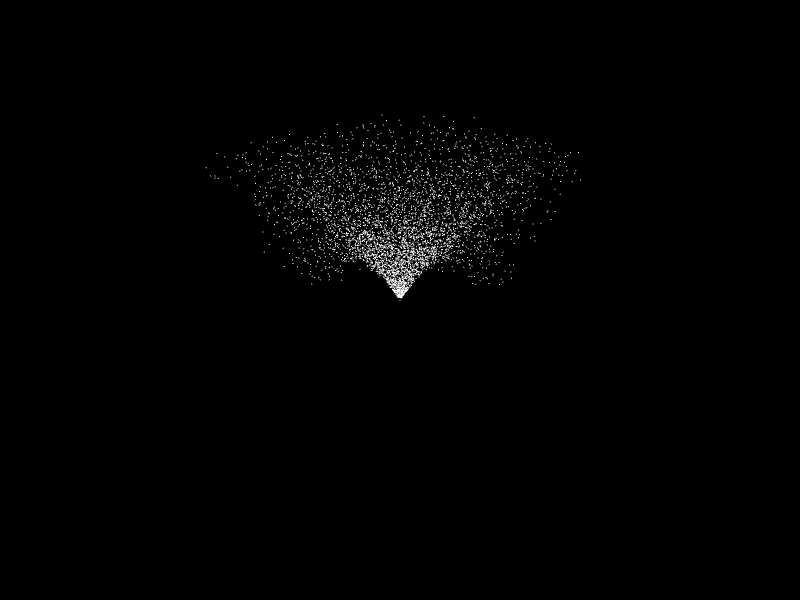### Enhancements

So, now we have ourselves a little fountain of white specks. What can we do to improve its appearance a bit?

#### Varying Particle Appearance With Age

The most obvious thing that comes to mind is to change up some stuff in the particle rendering shader based on how old a given particle is. I will vary three parameters: transparency, size, and color.

For this, we only need to update the code in particle rendering shaders. Here is the updated vertex shader for rendering a particle:

``````
#version 300 es
precision mediump float;

in vec2 i_Position;
in float i_Age;
in float i_Life;
in vec2 i_Velocity;

out float v_Age;
out float v_Life;

void main() {
/* Set varyings so that frag shader can use these values too.*/
v_Age = i_Age;
v_Life = i_Life;

/* Vary point size based on age. Make old particles shrink. */
gl_PointSize = 1.0 + 6.0 * (1.0 - i_Age/i_Life);

gl_Position = vec4(i_Position, 0.0, 1.0);
}
```
```

And here's the updated fragment shader:

``````
#version 300 es
precision mediump float;

in float v_Age;
in float v_Life;

out vec4 o_FragColor;

/* From http://iquilezles.org/www/articles/palettes/palettes.htm */
vec3 palette( in float t, in vec3 a, in vec3 b, in vec3 c, in vec3 d )
{  return a + b*cos( 6.28318*(c*t+d) ); }

void main() {
float t =  v_Age/v_Life;
o_FragColor = vec4(
palette(t,
vec3(0.5,0.5,0.5),
vec3(0.5,0.5,0.5),
vec3(1.0,0.7,0.4),
vec3(0.0,0.15,0.20)), 1.0 - t);
}
```
```

Here, I am once again borrowing this neat palette trick. Also, I am making older particles more transparent.

You will also have to make some adjustments to the javascript code for this to work. You'll have to add the following new members to the `render_attrib_locations` array in the `init` function:

``````
i_Age: {
location: gl.getAttribLocation(render_program, "i_Age"),
num_components: 1,
type: gl.FLOAT
},
i_Life: {
location: gl.getAttribLocation(render_program, "i_Life"),
num_components: 1,
type: gl.FLOAT
}
```
```

Here is what it looks like in action:

Click the image below to open an interactive demo in a new tab.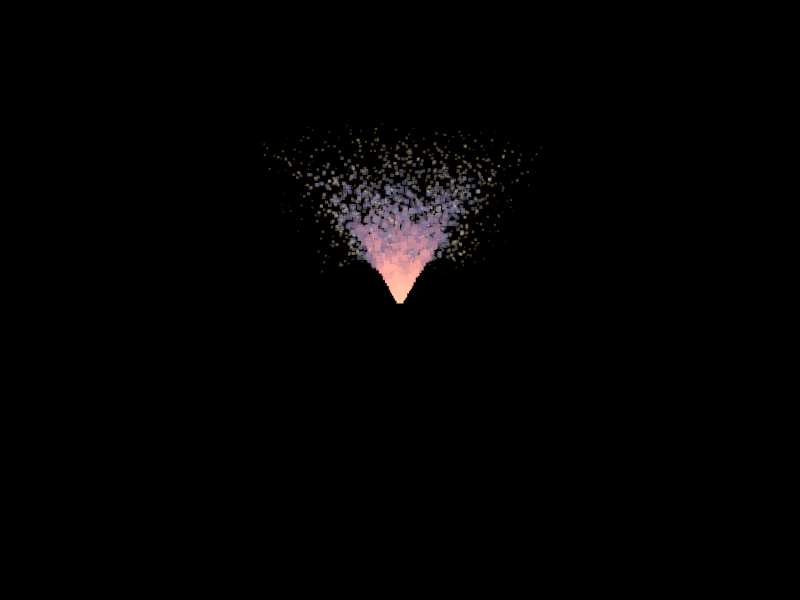#### Turning On the Force Field

Currently, there is one force affecting all of our particles at all times. We could add some interesting variation to the movement if we made different forces act on a particle depending on its position in space.

To that end, we're going to use an additional texture with red and green values. I'll refer to it as a "force field". Here's the one I'm going to use: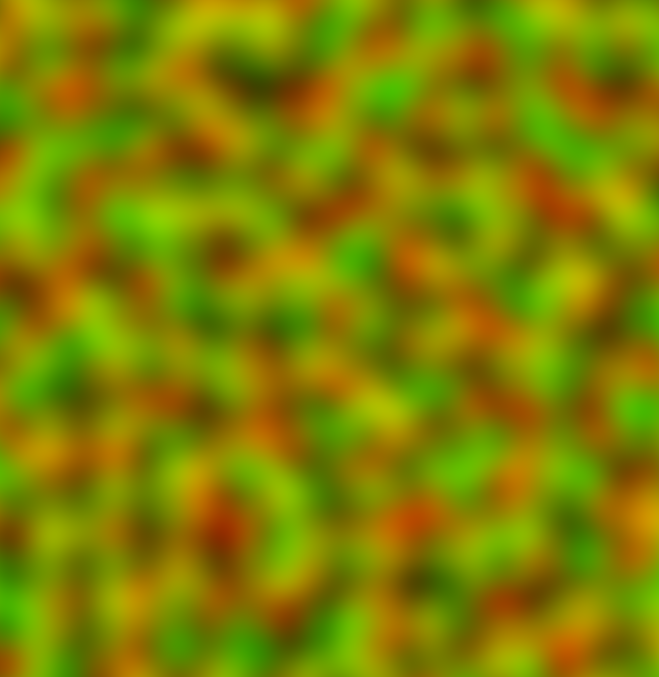This image simply has perlin noise in its red and green channels. I chose smooth continious noise because it results in more interesting movement.

The code will require a couple of modifications. The particle update shader receives a new uniform:

``````
//..
uniform sampler2D u_ForceField;
//..
```
```

It will also need to sample the force field like so:

``````
//...
vec2 force = 4.0 * (2.0 * texture(u_ForceField, i_Position).rg - vec2(1.0));
//...
```
```

And apply the force like this:

``````
//...
v_Velocity = i_Velocity + u_Gravity * u_TimeDelta + force * u_TimeDelta;
//...
```
```

The changes in the javascript code itself are trivial and pretty much just boil down to creating a new texture, uploading the noise image to it, and binding it before running the update step. Here's the result:

Click the image below to open an interactive demo in a new tab.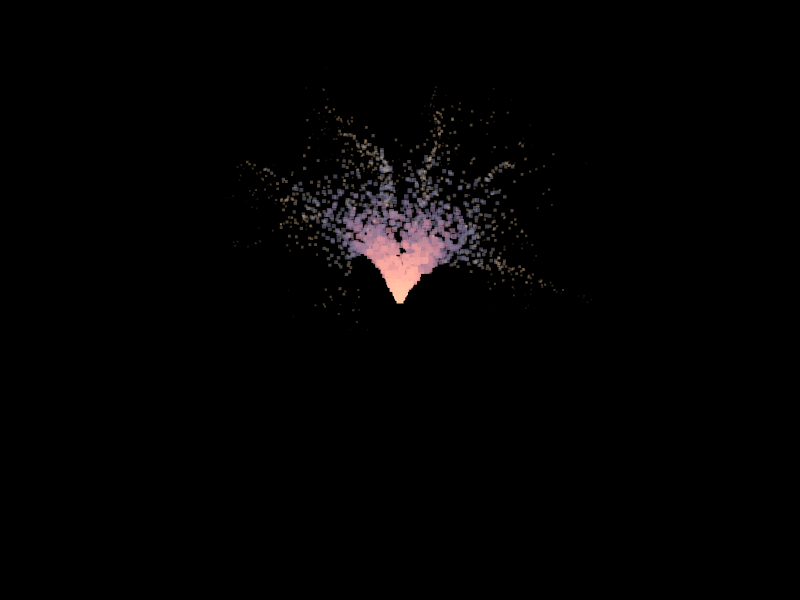That's nice, but can be taken further. If, instead of using a static texture, you actually generate Perlin noise in the fragment shader (as described here), and animate it with time, you can get something like this:

Click the image below to open an interactive demo in a new tab.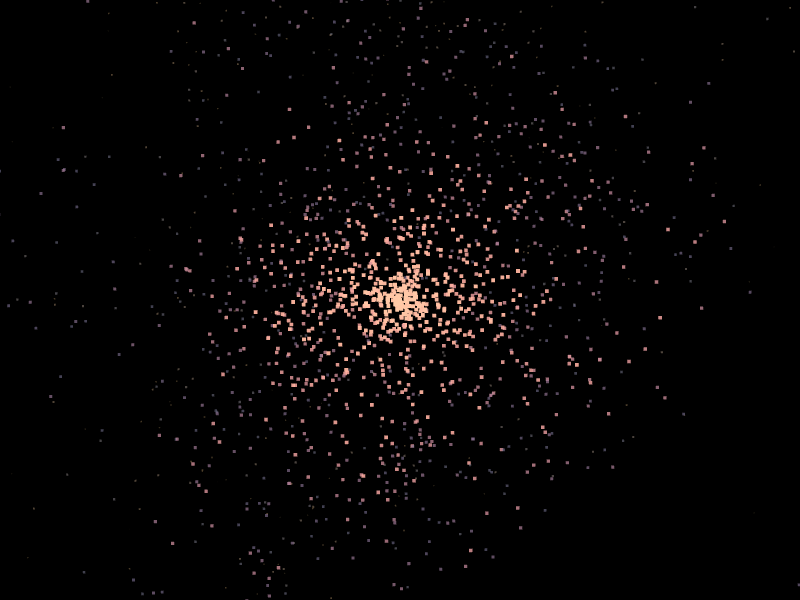#### Billboards

So far, we've been using point primitives to render our particles. Depending on the aesthetic you're going for, this might actually be perfectly suitable. However, in most cases, you probably want to draw something other than a point in place of each particle. For example, a textured quad.

This is where we run into some problems. Our particle data is per-vertex. So far, this hasn't been a problem - one point on screen corresponded to one vertex, and therefore, to one set of particle parameters. However, if we want to draw a quad, we need four vertices. So, we need a way of sharing a particle's parameters between the vertices of its corresponding quad.

One way of achieving this is using a geometry shader. The input to the geometry shader would be points, and it would generate corresponding quads on the other end. However, WebGL 2 does not support geometry shaders, so that option is right out.

Another way of doing it is instancing. Instancing allows you to render multiple instances of a mesh with a single draw call. When setting up your vertex arrays, you may choose some attributes to stay the same value within an instance (or even across a fixed number of consecutive instances). For example, you might have a "position" attribute, which only changes once per instance. For each instance, it would contain the world-space position at which to draw it.

We're going to do exactly that - our instances are going to be quads, while all the particle data (age, position, etc.) will be per-instance.

The particle update shader doesn't change at all.

There are some changes in the particle render shader though. Let's have a look at them. To make things easier, I've highlighted changed parts.

``````
#version 300 es
precision mediump float;

// These attributes stay the same for all vertices in an instance.
in vec2 i_Position;
in float i_Age;
in float i_Life;
in vec2 i_Velocity;

// These attributes change for each of the instance's vertices.
in vec2 i_Coord;
in vec2 i_TexCoord;

out float v_Age;
out float v_Life;
out vec2 v_TexCoord;

void main() {
float scale = 0.75; /* the quad is 1.0x1.0, we scale it appropriately */
vec2 vert_coord = i_Position +
(scale*(1.0-i_Age / i_Life) + 0.25) * 0.1 * i_Coord +
i_Velocity * 0.0;
v_Age = i_Age;
v_Life = i_Life;
v_TexCoord = i_TexCoord;
gl_Position = vec4(vert_coord, 0.0, 1.0);
}
```
```

As you can see, we've added new attributes for a quad's vertex positions and texture coordinates. The vertex positions will always be (-1,-1), (-1, 1), (1, 1), (1, -1). We'll translate and scale them based on particle attributes.

Let's have a quick look at the corresponding fragment shader as well.

``````
#version 300 es
precision mediump float;

// Particle sprite texture.
uniform sampler2D u_Sprite;

in float v_Age;
in float v_Life;
in vec2 v_TexCoord;

out vec4 o_FragColor;

void main() {
float t =  v_Age/v_Life;
vec4 color = vec4(vec3(1.0, 0.8, 0.3), 1.0-(v_Age/v_Life));
o_FragColor = color * texture(u_Sprite, v_TexCoord);
}
```
```

As you can see, we've added a sprite texture, and all this shader does is apply the texture to our quad.

The changes to the javascript code are a bit more substantial. First, let's have a look at the changes in the init function. I'll only show the relevant parts:

``````function init(
gl,
num_particles,
particle_birth_rate,
min_age,
max_age,
min_theta,
max_theta,
min_speed,
max_speed,
gravity,
part_img) { // Note the new parameter.
//...snip...
var render_attrib_locations = {
i_Position: {
location: gl.getAttribLocation(render_program, "i_Position"),
num_components: 2,
type: gl.FLOAT,
divisor: 1
},
i_Age: {
location: gl.getAttribLocation(render_program, "i_Age"),
num_components: 1,
type: gl.FLOAT,
divisor: 1
},
i_Life: {
location: gl.getAttribLocation(render_program, "i_Life"),
num_components: 1,
type: gl.FLOAT,
divisor: 1
}
};
var vaos = [
gl.createVertexArray(),
gl.createVertexArray(),
gl.createVertexArray(),
gl.createVertexArray()
];
var buffers = [
gl.createBuffer(),
gl.createBuffer(),
];
var sprite_vert_data =
new Float32Array([
1, 1,
1, 1,

-1, 1,
0, 1,

-1, -1,
0, 0,

1, 1,
1, 1,

-1, -1,
0, 0,

1, -1,
1, 0]);
var sprite_attrib_locations = {
i_Coord: {
location: gl.getAttribLocation(render_program, "i_Coord"),
num_components: 2,
type: gl.FLOAT,
},
i_TexCoord: {
location: gl.getAttribLocation(render_program, "i_TexCoord"),
num_components: 2,
type: gl.FLOAT
}
};
var sprite_vert_buf = gl.createBuffer();
gl.bindBuffer(gl.ARRAY_BUFFER, sprite_vert_buf);
gl.bufferData(gl.ARRAY_BUFFER, sprite_vert_data, gl.STATIC_DRAW);
var vao_desc = [
{
vao: vaos,
buffers: [{
buffer_object: buffers,
stride: 4 * 6,
attribs: update_attrib_locations
}]
},
{
vao: vaos,
buffers: [{
buffer_object: buffers,
stride: 4 * 6,
attribs: update_attrib_locations
}]
},
{
vao: vaos,
buffers: [{
buffer_object: buffers,
stride: 4 * 6,
attribs: render_attrib_locations
},
{
buffer_object: sprite_vert_buf,
stride: 4 * 4,
attribs: sprite_attrib_locations
}],
},
{
vao: vaos,
buffers: [{
buffer_object: buffers,
stride: 4 * 6,
attribs: render_attrib_locations
},
{
buffer_object: sprite_vert_buf,
stride: 4 * 4,
attribs: sprite_attrib_locations
}],
},
];
// ..snip..
gl.blendFunc(gl.SRC_ALPHA, gl.ONE);

var particle_tex = gl.createTexture();
gl.bindTexture(gl.TEXTURE_2D, particle_tex);
gl.texImage2D(gl.TEXTURE_2D, 0, gl.RGBA8, 32, 32, 0, gl.RGBA, gl.UNSIGNED_BYTE, part_img);
gl.texParameteri(gl.TEXTURE_2D, gl.TEXTURE_MIN_FILTER, gl.LINEAR);
gl.texParameteri(gl.TEXTURE_2D, gl.TEXTURE_MAG_FILTER, gl.LINEAR);
return {
//...snip...
particle_tex: particle_tex
};
}
```
```

Note the new "divisor" properties on attribute desriptions - these will be used by `setupParticleBufferVAO` to set the rate at which the value of these attributes changes (in this case, once per instance).

Of course, you will also need to call drawArraysInstanced instead of drawArrays in the rendering function.

And here it is in action, a blazing fireball:

Click the image below to open an interactive demo in a new tab.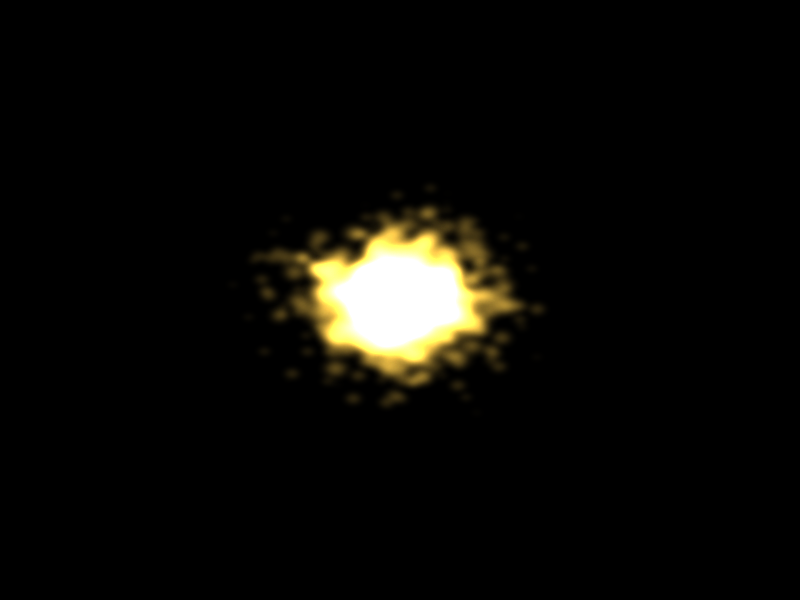And that'll be it for this post. This was fairly long, and code-heavy, so let me know if I can do a better job explaining some parts.